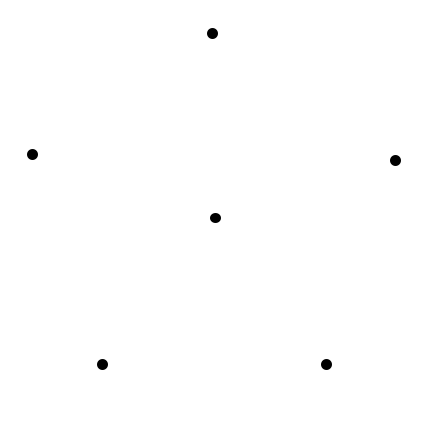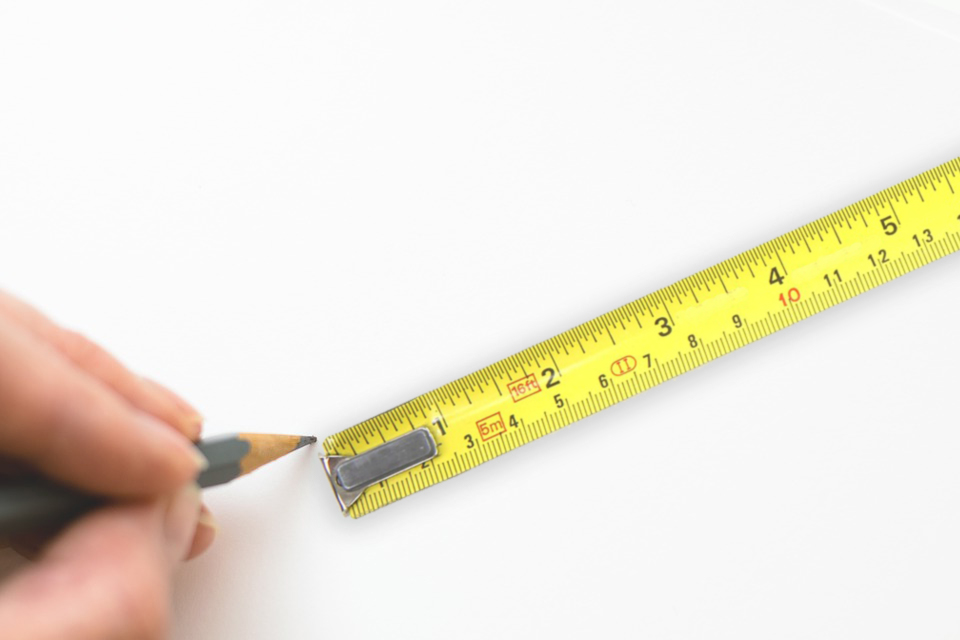## ________________

Points and lines are the theme this week.

## ________________Define a link as a straight line connecting any two points of the six points in the figure
to the right.

How many links can be placed without forming a triangle having three of the points
as vertices?### Seven Points

Place seven points at different positions on the same plane so that for any three chosen, at least two will be exactly one foot apart.

## Solutions to week 132

The time in Time of Day is 8:24 am. The simple continuous function in The Missing Element is F(x) = 120(2x – 1)/x. The terms shown are for x = -3, -2, -1, 0, 1, 2, 3, 4, 5, 6. q = F(0) = 120 × ln2 which is approximately equal to 83.17766.